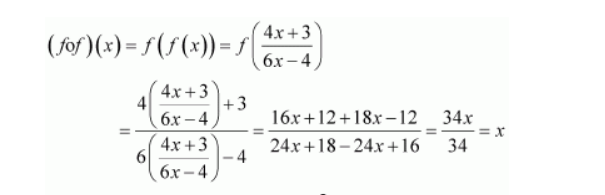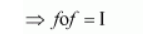# What is the inverse of f?`
Question:

If $f(x)=\frac{(4 x+3)}{(6 x-4)}, x \neq \frac{2}{3}$, show that $f \circ f(x)=x$, for all $x \neq \frac{2}{3} .$ What is the inverse of $f ?$

Solution:

It is given that $f(x)=\frac{(4 x+3)}{(6 x-4)}, x \neq \frac{2}{3}$.Therefore, $f \circ f(x)=x$, for all $x \neq \frac{2}{3}$.Hence, the given function f is invertible and the inverse of f is f itself.